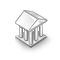Interesting script?
So post a link to it -
let others appraise it

You liked the script? Try it in the MetaTrader 5 terminal# VATicks - library for MetaTrader 4

Views:
5447
Rating:
Published:
2016.10.27 13:41

Class for working with ticks in the MetaTrader 4. It is suitable for creating experts that make decisions on trading based on the analysis of tick data.

The class can calculate:

• Price movement speed;
• Price acceleration;
• Distance passed by the price;
• Price movement direction;
• Distance the price may pass based on acceleration.

Example of using the class in an expert.

```#include <VATicks.mqh>

CTicks Tick;
int index;
int oldindex;
double V0;
//+------------------------------------------------------------------+
//| Expert initialization function                                   |
//+------------------------------------------------------------------+
int OnInit()
{
index = 15;
oldindex = 0;
V0 = 0;
return(INIT_SUCCEEDED);
}
//+------------------------------------------------------------------+
//| Expert deinitialization function                                 |
//+------------------------------------------------------------------+
void OnDeinit(const int reason)
{

}
//+------------------------------------------------------------------+
//| Expert tick function                                             |
//+------------------------------------------------------------------+
void OnTick()
{
Tick.Tick(Bid);
int count = Tick.TickCount();
if (count==index)
{
int T = Tick.TickTime(oldindex,count);
Print("Time for 15 ticks = ",T," s");
double S = Tick.Length(oldindex,count);
Print("Distance for 15 ticks = ",T," s");
double V = Tick.Speed(oldindex,count);
Print("Speed for 15 ticks = ",T," s");
double A = Tick.Acceleration(oldindex,count);
Print("Acceleration for 15 ticks = ",T," s");
double Sr = Tick.EstimatedPath(oldindex,count,V0);
Print("Can still pass (estimated distance based on acceleration) ",Sr," point ");
int tr=Tick.Trend(oldindex,count);
if (tr==TREND_BUY)  Print("Direction for 15 ticks is up");
if (tr==TREND_SELL)  Print("Direction for 15 ticks is down");
if (tr==TREND_NO)  Print("Direction for 15 ticks is none");
oldindex=index;
index+=16;
V0 = V;
}
}
```

Translated from Russian by MetaQuotes Software Corp.
Original code: https://www.mql5.com/ru/code/16154Cauchy derivative

Derivative of the Cauchy differenceCauchy difference

Cauchy difference.Indicator for monitoring the lot and profit

The indicator displays the total lot and profit for the current instrument.Price_Compare

Elegant and nimble comparison of 'double' values of the "price".